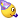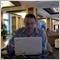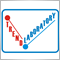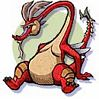# Moving Average - page 35214

New version(v2.2) of AllAverages is ready.

// List of MAs:

// MA_Method= 0: SMA - Simple Moving Average

// MA_Method= 1: EMA - Exponential Moving Average

// MA_Method= 2: Wilder - Wilder Exponential Moving Average

// MA_Method= 3: LWMA - Linear Weighted Moving Average

// MA_Method= 4: SineWMA - Sine Weighted Moving Average

// MA_Method= 5: TriMA - Triangular Moving Average

// MA_Method= 6: LSMA - Least Square Moving Average (or EPMA, Linear Regression Line)

// MA_Method= 7: SMMA - Smoothed Moving Average

// MA_Method= 8: HMA - Hull Moving Average by Alan Hull

// MA_Method= 9: ZeroLagEMA - Zero-Lag Exponential Moving Average

// MA_Method=10: DEMA - Double Exponential Moving Average by Patrick Mulloy

// MA_Method=11: T3 - T3 by T.Tillson

// MA_Method=12: ITrend - Instantaneous Trendline by J.Ehlers

// MA_Method=13: Median - Moving Median

// MA_Method=14: GeoMean - Geometric Mean

// MA_Method=15: REMA - Regularized EMA by Chris Satchwell

// MA_Method=16: ILRS - Integral of Linear Regression Slope

// MA_Method=17: IE/2 - Combination of LSMA and ILRS

// MA_Method=18: TriMAgen - Triangular Moving Average generalized by J.Ehlers

// MA_Method=19: VWMA - Volume Weighted Moving Average

// List of Prices:

// Price = 0 - Close

// Price = 1 - Open

// Price = 2 - High

// Price = 3 - Low

// Price = 4 - Median Price = (High+Low)/2

// Price = 5 - Typical Price = (High+Low+Close)/3

// Price = 6 - Weighted Close = (High+Low+Close*2)/4

// Price = 7 - Heiken Ashi Close

// Price = 8 - Heiken Ashi Open

// Price = 9 - Heiken Ashi High

// Price =10 - Heiken Ashi Low

As you can see 2 MAs and 4 prices(HeikenAshi) are added.

Moreover color mode is added too.

Cheers3203

New version(v2.2) of AllAverages is ready.

// List of MAs:

// MA_Method= 0: SMA - Simple Moving Average

// MA_Method= 1: EMA - Exponential Moving Average

// MA_Method= 2: Wilder - Wilder Exponential Moving Average

// MA_Method= 3: LWMA - Linear Weighted Moving Average

// MA_Method= 4: SineWMA - Sine Weighted Moving Average

// MA_Method= 5: TriMA - Triangular Moving Average

// MA_Method= 6: LSMA - Least Square Moving Average (or EPMA, Linear Regression Line)

// MA_Method= 7: SMMA - Smoothed Moving Average

// MA_Method= 8: HMA - Hull Moving Average by Alan Hull

// MA_Method= 9: ZeroLagEMA - Zero-Lag Exponential Moving Average

// MA_Method=10: DEMA - Double Exponential Moving Average by Patrick Mulloy

// MA_Method=11: T3 - T3 by T.Tillson

// MA_Method=12: ITrend - Instantaneous Trendline by J.Ehlers

// MA_Method=13: Median - Moving Median

// MA_Method=14: GeoMean - Geometric Mean

// MA_Method=15: REMA - Regularized EMA by Chris Satchwell

// MA_Method=16: ILRS - Integral of Linear Regression Slope

// MA_Method=17: IE/2 - Combination of LSMA and ILRS

// MA_Method=18:TriMAgen - Triangular Moving Average generalized by J.Ehlers

// MA_Method=19: VWMA - Volume Weighted Moving Average

// List of Prices:

// Price = 0 - Close

// Price = 1 - Open

// Price = 2 - High

// Price = 3 - Low

// Price = 4 - Median Price = (High+Low)/2

// Price = 5 - Typical Price = (High+Low+Close)/3

// Price = 6 - Weighted Close = (High+Low+Close*2)/4

// Price = 7 - Heiken Ashi Close

// Price = 8 - Heiken Ashi Open

// Price = 9 - Heiken Ashi High

// Price =10 - Heiken Ashi Low

As you can see 2 MAs and 4 prices(HeikenAshi) are added.

ha price (7-10):

haClose = (Open +High+Low+Close)/4;

haOpen = (Open[i+1]+Close)/2; (previous bar (Open+Close)/2)

haHigh = Max (High, Open, Close);

haLow = Min (Low, Open, Close) ;3203

Igor, why did you used mtf barshift f-la?

thank you140

Is there an indicator that gives an alert when price touches the moving average on a particular timeframe?

ThanksModerator
113446

Just found this post about MA Distance https://www.mql5.com/en/forum140

newdigital:53

Rolling MA

Anyone know where I can find the Rolling moving average indicator? Is it one of the MA's in the AllAverages v2.2 just with a different name? Someone please let me know. Thanks301

New version(v2.2) of AllAverages is ready.

// List of MAs:

// MA_Method= 0: SMA - Simple Moving Average

// MA_Method= 1: EMA - Exponential Moving Average

// MA_Method= 2: Wilder - Wilder Exponential Moving Average

// MA_Method= 3: LWMA - Linear Weighted Moving Average

// MA_Method= 4: SineWMA - Sine Weighted Moving Average

// MA_Method= 5: TriMA - Triangular Moving Average

// MA_Method= 6: LSMA - Least Square Moving Average (or EPMA, Linear Regression Line)

// MA_Method= 7: SMMA - Smoothed Moving Average

// MA_Method= 8: HMA - Hull Moving Average by Alan Hull

// MA_Method= 9: ZeroLagEMA - Zero-Lag Exponential Moving Average

// MA_Method=10: DEMA - Double Exponential Moving Average by Patrick Mulloy

// MA_Method=11: T3 - T3 by T.Tillson

// MA_Method=12: ITrend - Instantaneous Trendline by J.Ehlers

// MA_Method=13: Median - Moving Median

// MA_Method=14: GeoMean - Geometric Mean

// MA_Method=15: REMA - Regularized EMA by Chris Satchwell

// MA_Method=16: ILRS - Integral of Linear Regression Slope

// MA_Method=17: IE/2 - Combination of LSMA and ILRS

// MA_Method=18: TriMAgen - Triangular Moving Average generalized by J.Ehlers

// MA_Method=19: VWMA - Volume Weighted Moving Average

// List of Prices:

// Price = 0 - Close

// Price = 1 - Open

// Price = 2 - High

// Price = 3 - Low

// Price = 4 - Median Price = (High+Low)/2

// Price = 5 - Typical Price = (High+Low+Close)/3

// Price = 6 - Weighted Close = (High+Low+Close*2)/4

// Price = 7 - Heiken Ashi Close

// Price = 8 - Heiken Ashi Open

// Price = 9 - Heiken Ashi High

// Price =10 - Heiken Ashi Low

As you can see 2 MAs and 4 prices(HeikenAshi) are added.

Moreover color mode is added too.

thanks igorad,,maybe next version will be have BAR version with all moving average6936

It's so-called Wilder smoothing(MA_Method=2).

Wilder(or RMA) = MA + 1/N*(P - MA)

where

P -price

N -Length(Period) of the Moving Average

TheDirtySerb:
Anyone know where I can find the Rolling moving average indicator? Is it one of the MA's in the AllAverages v2.2 just with a different name? Someone please let me know. Thanks21

MA text

Is there an indicator that presents MA's in text form?

Tks